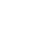# Continuous Bag of Words (CBOW) – Multi Word Model – How It Works

This post is an extension of single word continuous bag of words (CBOW) model, where I have discussed how CBOW model works for single word to understand Continuous Bag of Word (CBOW) clearly.
In single word CBOW model it was easy to prepare data and train that as there was only one input (context) word and one output (target) word. But for multi-word model there will be multiple words as input. As shown in the below picture.

Here we have taken window size = 1, but we are considering window to the both direction, unlike single word model (unidirectional window).

# Continuous Bag of Words (CBOW) multi-word model:

In this section we will be implementing the CBOW for multi-word architecture of Word2Vec. Like single word CBOW, the content is broken down into the following steps:
Data Preparation: Defining corpus by tokenizing text.
Generate Training Data: Build vocabulary of words, one-hot encoding for words, word index.
Train Model: Pass one hot encoded words through forward pass, calculate error rate by computing loss, and adjust weights using back propagation.
Output: By using trained model calculate word vector and find similar words.
I will explain CBOW steps without code but if you want full working code of CBOW with numpy from scratch, I have separate post for that, you can always jump into that.

## 1. Data Preparation for CBOW:

Let’s say we have a text like below:
i like natural language processing
To make it simple I have chosen a sentence without capitalization and punctuation. Also I will not remove any stop words (“and”, “the” etc.) but for real world implementation you should do lots of cleaning task like stop word removal, replacing digits, remove punctuation etc.
After pre-processing we will convert the text to list of tokenized word.
Output:
[“i”, “like”, “natural”, “language”, “processing”]

## 2. Generate training data for CBOW:

Unique vocabulary: Find unique vocabulary list. Here we don’t have any duplicate word in our example text, so unique vocabulary will be:
[“i”, “like”, “natural”, “language”, “processing”]
Now to prepare training data for single word CBOW model, we define “target word” as the word which follows a given word in the text (which will be our “context word”). That means we will be predicting next word for a given word.
Now let’s construct our training examples, scanning through the text with a window will prepare a context word and a target word, like so:
For example, for context word “i” and “natural” the target word will be “like”. For our example text full training data will looks like:
One-hot encoding: We need to convert text to one-hot encoding as algorithm can only understand numeric values.
For example encoded value of the word “i”, which appears first in the vocabulary, will be as the vector [1, 0, 0, 0, 0]. The word “like”, which appears second in the vocabulary, will be encoded as the vector [0, 1, 0, 0, 0]
So let’s see overall set of context-target words in one hot encoded form:
So as you can see above table is our final training data, where encoded target word is Y variablefor our model and encoded context word is X variable for our model.
Now we will move on to train our model.

## 3. Training Multi word CBOW Model:

Multi-Word CBOW Model with window-size = 1
Now these multiple context words need to be converted into one word/vector so that we can feed these into neural network model. This is where multi-word CBOW differs from single-word CBOW.
To do this we will just take mean of those multiple context word (one-hot-encoded vector). Apart from that everything to train model and update weight will be as single word continuous bag of words (CBOW).
So now our final training data should looks like below:
Now we are done with our final training.
Rest training and updating weights process will be same as single-word CBOW model.

# Conclusion:

Now to conclude with listing all generalized equations. Here we just need to replace x with x-bar(mean value of x or context word) from single word CBOW model. Apart from that everything will be same.

## Forward Propagation:

\\\mathbf{h = \overline{x}\ u = w^{'}h}\\\\ \mathbf{y_j = softmax(u_j)=\frac{e^{j}}{\sum_{j=1}^{V}e^{j}}}\\\\ \mathbf{E=-log(w_t|w_c)=-u_{j^{*}}+log\sum_{j=1}^{V}e^{u_j}}

## Back Propagation:

### Update second weight (w/11):

\\\frac{dE}{dw^{'}}=e*h\\ So,\ new(dw^{'}_{11})=dw^{'}_{11}-\frac{dE(y_1)}{dw^{'}_{11}}\\ =w^{'}_{11}-e_1h_1

### General Vector Notation:

\\\frac{dE}{dw^{'}}=(w\overline{x})\otimes e\\\\ new(w^{'})=w^{'}_{old}-\frac{dE}{dw^{'}}

Note:Symbol denotes the outer product. In Python this can be obtained using the numpy.outer method

### Update second weight (w11):

\mathbf{\frac{dE}{dw_{11}}=(ew^{'}_{11}+ew^{'}_{12}+ew^{'}_{13}+ew^{'}_{14}+ew^{'}_{15})*\overline{x}}

### General Vector Notation:

\\\frac{dE}{dw}=\overline{x}\otimes (w^{'}e)\\\\ new(w)=w_{old}-\frac{dE}{dw}

Note:Symbol denotes the outer product. In Python this can be obtained using the numpy.outer method
If you have any question or suggestion regarding this topic see you in comment section. I will try my best to answer.

### 1 thought on “Continuous Bag of Words (CBOW) – Multi Word Model – How It Works”

1.Great Explanation

Automated page speed optimizations for fast site performance